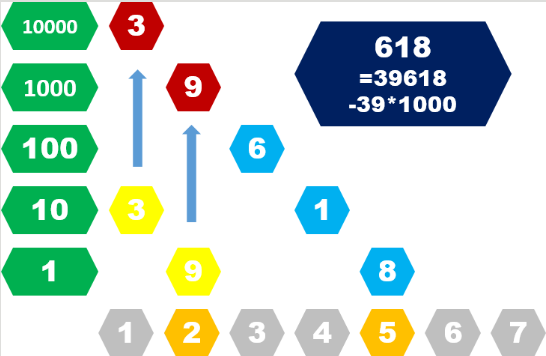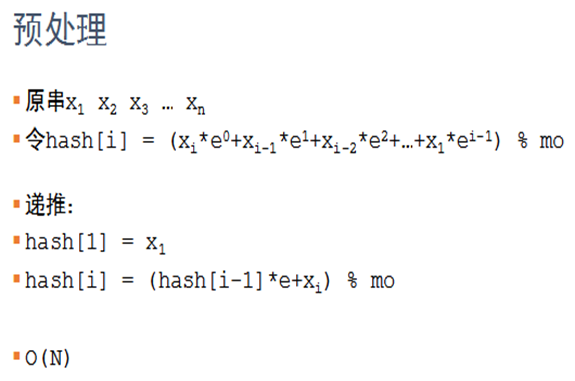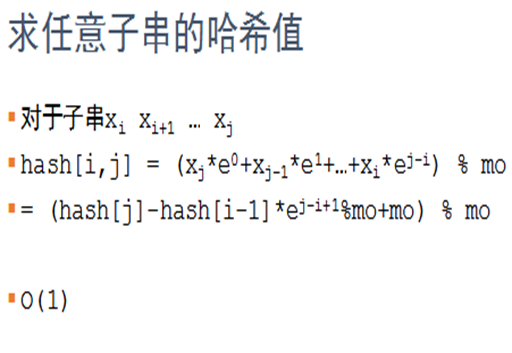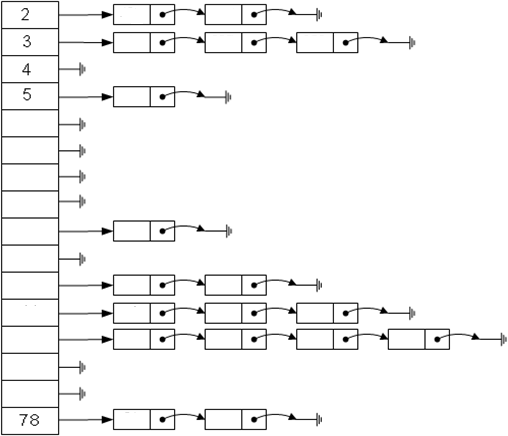# 学术上的问题 - 关于哈希``` 1 string s; // s 为字符串
2 int f[N], g[N]; // f 为前缀和，g[i] 为 D 的 i 次方
3 void prehash(int n) // 预处理哈希值
4 {// 预处理时，注意到数字可能很大，对一个数 MD 取模
5     f = s; // f 前缀和预处理
6     for(int i=1; i<=n; i++)
7     {
8         f[i] = (1LL * f[i-1] * D + s[i-1]) % MD;
9     }
10     g = 1; // g：D 次方预处理
11     for(int i=1; i<=n; i++)
12     {
13         g[i] = 1LL * g[i-1] * D % MD;
14     }
15 }
16 int hash(int l, int r) // 计算区间 [l,r] 的哈希值
17 {
18     int a = f[r];
19     int b = 1LL * f[l-1] * g[r-l+1] % MD; // 记得乘上次方
20     return (a - b + MD) % MD; // 前缀和相减
21 // 有可能结果小于 0，加上一个 MD 将其变为正数
22 }
23     if(hash(a, b) == hash(c, d)) // 字符串 [a,b] 与字符串 [c,d] 匹配```

1、拉链法（链地址法）：核心代码如下：

``` 1 //链地址法
2 vector<int> hash_array[N];
3 // hash_array：每个位置用一个 vector 来维护
4 void push2(int x)
5 {
6     int y = x % N; // 计算初始位置
7     for(int i=0; i<hash_array[y].size(); i++)  if(hash_array[y][i] == x) // 如果之前已经出现过了
8     {
9         cout << x << "?has?occured?before!" << endl;
10         return; // 标记已经出现过
11     }
12 // 如果之前没有出现过，将 x 加入表中
13     hash_array[y].push_back(x);
14     cout << x << "?inserted." << endl;
15 }```

2.顺序询址法：

```//顺序寻址法
int hash_table[N]; // hash_table 哈希表：0 位置代表没有数
void push1(int x)
{
int y = x % N; // 计算初始位置，N：表的大小
for(; hash_table[y]!=0 && hash_table[y]!=x; ) y = (y+1) % N;
// 寻找到一个 0 位置，或者找到自己为止
if(hash_table[y]) cout << x << "?has?occured?before!" << endl;
// 如果是自己本身，则之前已经出现过了
else
{
hash_table[y] = x; // 否则，将 x 加入表中
cout << x << "?inserted." << endl;
}
}```

```#include<cstdio>
#include<iostream>
using namespace std;
const int N=50000; //定义总共输入哈希数字个数
const int b=999979,H=999979;//哈希取模%数字
int top,stk[N];
void init()
{ //初始化哈希表
tot=0;
while(top)  //我们用一个栈存储下出现过的哈希值
}    //每次把出现过的哈希值的链表清0，来节省时间

void insert(int key)
{  //将一个数字插入哈希表
int h=key%b; //除余法
if(num[e]==key) //诺链表中已存在当前数字则不再存
return;
num[tot]=key; //建立链接表，存储值等于key的数字。
}

bool query(int key)
{
int h=key%b;
if(num[e]==key) return true;
return false;
}

int main()
{
int a;
init();
int n,m;
cin>>n;
for(int i=1;i<=n;++i)
{
cin>>a[i];
insert(a[i]);
}
cin>>m;
int num;
for(int i=1;i<=m;++i)
{
cin>>num;
if(query(num))
printf("yes\n");
else
printf("no\n");
}
}`````` 1 #include<cstdio>
2 #include<iostream>
3 #include<algorithm>
4 #include<cstring>
5 #define ull unsigned long long
6 using namespace std;
7 const int N=1e4+1;
8 char c[N];
9 ull a[N];
10 ull num=131;
11 int ans,n;
12 int prime=233317;
13 ull mod=212370440130137957ll;
14 ull hasha(char s[])
15 {
16     int len=strlen(c);
17     ull ans=0;
18     for(int i=1;i<=len;i++)
19     {
20         ans=(ans*num+(ull)c[i])%mod+prime;
21     }
22     return ans;
23 }
24 int main()
25 {
26     int n;
27     cin>>n;
28     for(int i=1;i<=n;i++)
29     {
30         cin>>c;
31         a[i]=hasha(c);
32     }
33     sort(a+1,a+n+1);
34     for(int i=1;i<n;i++)
35     {
36         if(a[i]!=a[i+1])
37         {
38             ans++;
39         }
40     }
41     cout<<ans+1;
42     return 0;
43 }```